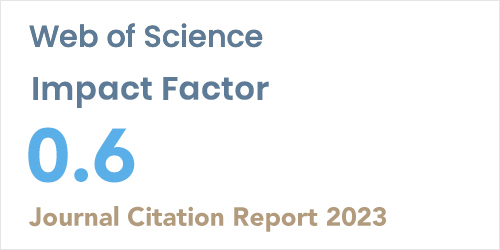ISSN: 1304-7191 | E-ISSN: 1304-7205
Volume: 41 Issue: 5 Year: 2023Approximate solutions of the fractional harry DYM equation
1Suleyman Demirel University, Department of Avionics, Isparta, Türkiye
Sigma J Eng Nat Sci - DOI: 10.14744/sigma.2023.00073

#### Abstract

In this paper, the approximate solutions of the time fractional Harry Dym equation with fractional derivative in the Caputo sense are obtained by using the Residual power series method (RPSM). This equation is a significant dynamical equation that occurs in a variety of physical systems. The suggested method provides good accuracy for the approximate solution when compared numerically with the exact solution. The effectiveness of the proposed method is also illustrated with the aid of numerical results. These results indicate that the RPSM is a power, useful, and applicable for determining the solutions of the time Hary Dym equation. Some of these results are illustrated by 2D and 3D graphics. Besides, the proposed method can be applied to many different differential equations due to its ease of use and reliability.